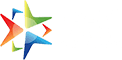Download Subscription Form

# Publish a Book Chapter in "Research Trends in Mathematics and Statistics (Volume - 24)"

## Mathematics Edited Book | Edited Book on Mathematics

This edited book on mathematics titled "Research Trends in Mathematics and Statistics" mainly focuses on various topics such as algebra, geometry, linear and multilinear algebra etc., and the rest are given below in the Scope of the book. This mathematics edited book will be published with ISBN and DOI numbers after following a proper double blind peer reviewed process. All the chapters of this mathematics edited book will be published in a very illustrative manner for easy reader understanding.Book Scope

• Algebra
• Geometry
• Linear and multilinear algebra; matrix theory
• Real functions
• Measure and integration
• Functions of a complex variable
• Several complex variables and analytic spaces
• Ordinary differential equations
• Partial differential equations
• Dynamical systems and ergodic theory
• Difference and functional equations
• Fourier analysis
• Integral equations
• Functional analysis
• Operator theory
• Calculus of variations and optimal
• control; optimization
• Operations research
• mathematical programming
• Differential geometry
• General topology
• Manifolds and cell complexes
• Global analysis
• analysis on manifolds
• Probability theory and stochastic processes
• Statistics
• Numerical analysis
• Computer science
• Mechanics of particles and systems
• Mechanics of deformable solids
• Fluid mechanics
• Optics
• electromagnetic theory
• Quantum theory
• Statistical mechanics

Author Guidelines

### Chief EditorDr. Arun Kumar Bharti

Assistant Professor, Dept. of Statistics, Meerut College, Meerut, Uttar Pradesh, India

### Co-Editor(s)

Dr. V.K. Tripathi

Assistant Professor (Mathematics), Department of Mathematics, Directorate of Distance Education, GJUS & T, Hisar, Haryana, IndiaDr. Savita Deswal

Associate Professor, Government P.G. College for Women, Rohtak, Haryana, India

How To Submit

Email your book chapter at akinikbooks@gmail.com

Customer helpline number +91-9911215212, +91-9999779515